# Algebra 1 : How to solve absolute value equations

## Example Questions

1 2 3 4 5 6 7 9 Next →

### Example Question #81 : How To Solve Absolute Value Equations

Solve for x: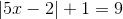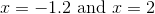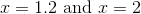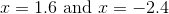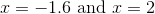Explanation:Subtract 1 from both sides: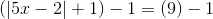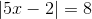Remove absolute Value Signs.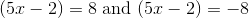Solve for both solutions of x: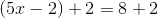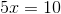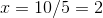AND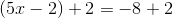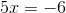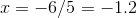### Example Question #82 : How To Solve Absolute Value Equations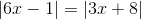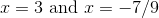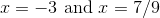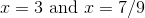Explanation:Removing the absolute value signs we get the four equations: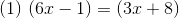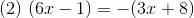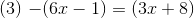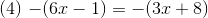Since 1&4 are identical and 2&3 are identical, we only need to solve for (1) and (2) to get the complete answer:

Equation 1 solution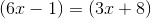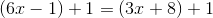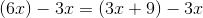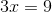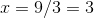Equation 2 Solution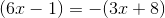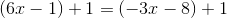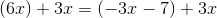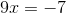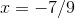1 2 3 4 5 6 7 9 Next →